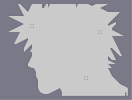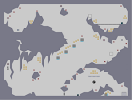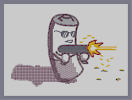### FarewellHover over the thumbnail for a full-size version.

Author ]{NO3 author:]{no3 boba fett n-art nonplayable rated starwars 2009-03-19 2012-04-21 5 by 64 people. \$Farewell#]{NO3#n-art#00000000000000000000000000000000000000000000000000000000000000000000000000000000000000000000000000000000000000000000000000000000000000000000000000000000000000000000000000000000000000000000000000000000000000000000000000000000000000000000000000000000000000000000000000000000000000000000000000000000000000000000000000000000000000000000000000000000000000000000000000000000000000000000000000000000000000000000000000000000000000000000000000000000000000000000000000000000000000000000000000000000000000000000000000000000000000000000000000000000000000000000000000000000000000000000000000000000000000000000000000000000000000000000000000000000000000000000000000000000000000000000000000000000000000000000000000000000000000000|12^135,310!12^140,309!12^144,306!12^147,303!12^152,301!12^800,605!12^156,299!12^162,297!12^167,293!12^171,290!12^174,289!12^179,286!12^183,284!12^189,281!12^194,278!12^199,275!12^203,273!12^209,270!12^213,267!12^218,266!12^225,263!12^230,261!12^234,261!12^240,258!12^244,255!12^249,253!12^253,251!12^258,250!12^262,247!12^266,246!12^271,243!12^277,241!12^282,239!12^287,237!12^291,234!12^297,233!12^301,232!12^305,230!12^309,228!12^311,226!12^315,226!12^319,225!12^322,223!12^325,221!12^327,221!12^332,219!12^337,217!12^341,215!12^343,214!12^347,213!12^351,211!12^355,211!12^360,210!12^365,209!12^369,207!12^373,206!12^379,205!12^383,203!12^388,202!12^393,201!12^397,201!12^401,201!12^405,199!12^410,198!12^414,197!12^417,195!12^405,199!12^409,198!12^413,197!12^420,195!12^422,195!12^424,197!12^430,195!12^430,199!12^425,201!12^419,202!12^417,203!12^411,206!12^407,208!12^404,209!12^399,210!12^397,212!12^141,322!12^142,319!12^141,314!12^146,319!12^151,319!12^155,318!12^159,317!12^163,317!12^167,317!12^172,317!12^177,316!12^181,315!12^185,315!12^190,315!12^194,315!12^199,315!12^205,315!12^209,315!12^213,314!12^219,315!12^220,318!12^221,322!12^222,325!12^223,329!12^226,332!12^227,336!12^229,340!12^230,343!12^231,347!12^233,351!12^235,354!12^235,358!12^237,361!12^238,364!12^239,368!12^242,372!12^243,376!12^245,379!12^247,385!12^249,389!12^250,393!12^251,397!12^253,402!12^254,405!12^258,408!12^256,410!12^258,414!12^259,417!12^262,421!12^262,425!12^263,429!12^265,433!12^265,437!12^266,441!12^268,444!12^270,447!12^270,450!12^272,453!12^273,457!12^275,459!12^277,463!12^278,465!12^280,469!12^281,473!12^282,478!12^284,481!12^286,483!12^287,486!12^289,490!12^289,494!12^291,497!12^293,501!12^293,505!12^294,508!12^295,513!12^297,516!12^298,518!12^299,522!12^301,525!12^303,527!12^303,529!12^393,214!12^390,216!12^386,217!12^381,218!12^378,220!12^375,221!12^372,223!12^369,225!12^365,226!12^362,227!12^359,229!12^357,230!12^354,231!12^350,233!12^347,234!12^345,235!12^341,237!12^337,239!12^333,240!12^330,242!12^327,244!12^325,246!12^321,248!12^318,250!12^315,252!12^313,254!12^310,256!12^307,257!12^304,259!12^301,261!12^298,263!12^294,265!12^291,267!12^289,269!12^285,271!12^283,274!12^281,276!12^277,279!12^277,280!12^277,284!12^278,288!12^279,291!12^280,294!12^281,297!12^283,300!12^284,303!12^285,307!12^286,310!12^287,313!12^289,315!12^290,318!12^291,321!12^293,323!12^294,327!12^295,331!12^296,334!12^298,337!12^299,340!12^299,343!12^301,346!12^302,350!12^303,353!12^303,354!12^303,358!12^304,363!12^306,367!12^307,369!12^305,359!12^306,363!12^309,367!12^310,372!12^310,376!12^313,379!12^314,383!12^315,386!12^317,390!12^317,394!12^318,397!12^321,401!12^322,405!12^323,409!12^325,413!12^326,416!12^327,419!12^329,423!12^330,427!12^330,427!12^369,516!12^367,513!12^365,510!12^363,508!12^362,505!12^361,502!12^359,499!12^358,495!12^356,493!12^354,490!12^353,485!12^353,482!12^352,478!12^350,475!12^349,473!12^347,470!12^346,467!12^343,463!12^342,459!12^341,456!12^340,452!12^339,449!12^338,445!12^335,442!12^334,439!12^332,435!12^331,433!12^330,429!12^330,428!12^383,510!12^383,506!12^382,503!12^381,500!12^379,497!12^378,491!12^375,488!12^374,485!12^373,481!12^371,477!12^371,475!12^391,261!12^389,262!12^385,265!12^383,267!12^382,270!12^379,273!12^377,275!12^374,279!12^372,282!12^370,285!12^369,289!12^366,293!12^365,295!12^363,298!12^362,302!12^359,306!12^357,310!12^357,315!12^356,318!12^355,322!12^355,326!12^354,329!12^353,334!12^353,339!12^353,343!12^352,348!12^351,352!12^351,357!12^350,358!12^351,327!12^351,330!12^350,336!12^349,341!12^349,346!12^349,350!12^347,355!12^346,359!12^345,366!12^344,373!12^344,377!12^344,381!12^346,386!12^349,390!12^351,394!12^352,398!12^352,398!12^347,391!12^348,396!12^349,401!12^349,405!12^351,408!12^352,412!12^353,415!12^354,419!12^355,423!12^356,427!12^357,431!12^359,434!12^361,438!12^362,442!12^363,446!12^365,450!12^365,455!12^366,459!12^368,461!12^370,465!12^370,469!12^371,473!12^395,258!12^399,256!12^403,253!12^406,251!12^409,249!12^413,247!12^416,245!12^419,244!12^423,244!12^429,241!12^432,239!12^436,238!12^440,237!12^445,235!12^449,235!12^450,238!12^451,241!12^451,246!12^451,250!12^451,254!12^451,258!12^450,263!12^449,267!12^447,272!12^446,277!12^445,282!12^443,289!12^443,294!12^442,299!12^441,302!12^439,306!12^434,309!12^431,311!12^428,312!12^423,314!12^419,318!12^413,319!12^410,320!12^406,323!12^403,325!12^390,498!12^386,494!12^383,489!12^383,485!12^383,479!12^383,474!12^383,470!12^382,465!12^381,461!12^380,457!12^378,453!12^378,449!12^377,447!12^387,492!12^387,487!12^385,484!12^382,481!12^379,474!12^402,329!12^397,333!12^394,337!12^392,341!12^389,345!12^387,349!12^386,353!12^382,361!12^381,365!12^381,369!12^380,372!12^379,375!12^378,382!12^375,388!12^374,393!12^374,397!12^374,401!12^374,406!12^374,410!12^374,418!12^374,425!12^374,430!12^374,435!12^374,439!12^375,443!12^378,445!12^394,502!12^397,501!12^401,499!12^405,498!12^409,495!12^414,493!12^417,489!12^419,485!12^421,482!12^423,480!12^427,478!12^433,474!12^437,472!12^440,469!12^444,466!12^449,462!12^453,458!12^455,455!12^458,452!12^461,449!12^464,447!12^468,444!12^470,441!12^473,438!12^478,435!12^481,433!12^484,430!12^487,426!12^492,421!12^494,418!12^498,415!12^501,411!12^504,407!12^507,404!12^510,400!12^514,395!12^516,393!12^521,391!12^523,388!12^523,388!12^126,297!12^124,299!12^124,303!12^124,307!12^124,311!12^126,315!12^127,318!12^129,322!12^131,325!12^132,328!12^133,332!12^134,336!12^135,340!12^137,343!12^139,347!12^142,350!12^145,353!12^147,354!12^150,355!12^154,356!12^158,356!12^162,357!12^166,357!12^170,358!12^174,360!12^178,361!12^182,363!12^185,365!12^186,365!12^190,368!12^193,370!12^195,373!12^198,375!12^201,377!12^204,379!12^209,383!12^211,385!12^213,387!12^215,390!12^217,392!12^221,395!12^223,398!12^225,401!12^227,405!12^229,408!12^231,411!12^233,415!12^236,419!12^241,425!12^243,430!12^245,432!12^246,434!12^239,423!12^239,423!12^288,530!12^286,525!12^285,522!12^284,518!12^283,515!12^282,512!12^281,509!12^279,505!12^278,501!12^277,498!12^275,494!12^274,490!12^273,487!12^270,484!12^269,481!12^267,479!12^266,476!12^265,472!12^263,468!12^262,465!12^260,462!12^258,459!12^255,456!12^254,451!12^252,449!12^250,445!12^248,441!12^247,439!12^196,523!12^200,523!12^204,524!12^207,525!12^211,525!12^217,526!12^221,527!12^224,528!12^228,528!12^232,528!12^235,529!12^240,529!12^246,529!12^249,529!12^253,529!12^257,530!12^263,530!12^267,530!12^271,530!12^275,531!12^280,531!12^284,531!12^217,245!12^222,245!12^229,242!12^229,242!12^225,242!12^228,241!12^231,239!12^235,238!12^239,237!12^243,235!12^247,234!12^251,233!12^255,231!12^259,229!12^263,227!12^266,226!12^269,225!12^275,222!12^279,220!12^283,219!12^287,217!12^290,215!12^295,214!12^299,213!12^305,211!12^309,209!12^313,207!12^317,205!12^321,204!12^324,202!12^329,201!12^333,199!12^337,197!12^341,196!12^345,195!12^349,194!12^353,193!12^356,192!12^360,191!12^363,189!12^367,189!12^370,187!12^374,185!12^378,184!12^381,182!12^384,182!12^388,181!12^391,180!12^397,179!12^400,178!12^404,177!12^408,176!12^411,175!12^415,174!12^420,172!12^426,172!12^430,172!12^430,172!12^463,153!12^459,148!12^456,145!12^454,141!12^453,137!12^451,134!12^450,130!12^447,127!12^445,123!12^443,118!12^441,115!12^439,112!12^435,107!12^434,105!12^431,102!12^427,99!12^424,95!12^421,93!12^415,88!12^300,54!12^304,54!12^307,53!12^311,53!12^315,53!12^320,52!12^324,52!12^328,52!12^333,52!12^338,53!12^343,55!12^347,56!12^352,57!12^355,58!12^361,59!12^365,61!12^369,62!12^373,63!12^375,65!12^379,67!12^383,69!12^387,71!12^391,74!12^397,75!12^402,77!12^405,80!12^410,82!12^411,83!12^141,154!12^144,150!12^146,147!12^147,144!12^149,141!12^150,138!12^153,135!12^155,133!12^158,130!12^159,127!12^162,123!12^163,120!12^166,118!12^167,115!12^170,113!12^173,110!12^177,107!12^181,105!12^183,103!12^186,101!12^190,98!12^194,96!12^197,93!12^199,91!12^203,89!12^207,86!12^212,83!12^217,81!12^221,79!12^223,78!12^226,75!12^230,74!12^234,73!12^239,73!12^243,70!12^246,69!12^250,68!12^255,66!12^262,64!12^266,63!12^272,61!12^277,60!12^282,58!12^286,57!12^292,56!12^294,55!12^125,210!12^124,206!12^125,203!12^125,199!12^127,195!12^129,190!12^130,186!12^131,183!12^132,178!12^133,175!12^134,170!12^136,167!12^138,162!12^139,158!12^139,157!12^125,216!12^125,220!12^125,224!12^123,227!12^123,231!12^123,236!12^125,243!12^126,247!12^126,251!12^125,256!12^125,260!12^125,265!12^125,271!12^125,281!12^127,284!12^128,287!12^129,287!12^127,292!12^127,292!12^123,274!12^123,274!12^116,278!12^108,279!12^107,283!12^107,287!12^109,291!12^110,298!12^113,302!12^114,307!12^115,313!12^116,318!12^118,323!12^120,327!12^123,331!12^125,336!12^126,341!12^127,345!12^129,349!12^129,353!12^130,358!12^132,360!12^132,362!12^133,367!12^135,373!12^137,377!12^138,381!12^139,385!12^141,390!12^143,395!12^145,400!12^146,405!12^147,409!12^149,413!12^150,419!12^153,423!12^154,428!12^161,446!12^163,450!12^165,455!12^166,458!12^168,462!12^169,463!12^160,441!12^158,438!12^158,434!12^156,431!12^156,431!12^569,393!12^573,393!12^577,391!12^581,390!12^586,390!12^591,390!12^596,390!12^602,392!12^606,393!12^611,393!12^615,393!12^619,393!12^624,394!12^631,394!12^642,395!12^647,395!12^652,395!12^658,395!12^663,395!12^669,394!12^699,392!12^699,391!12^696,390!12^692,390!12^687,390!12^683,390!12^678,390!12^674,392!12^671,392!12^701,390!12^706,387!12^711,386!12^718,385!12^724,383!12^730,382!12^738,382!12^745,382!12^751,382!12^763,384!12^767,385!12^767,385!12^758,384!12^754,384!12^754,384!12^734,381!12^734,381!12^699,395!12^696,398!12^694,401!12^693,404!12^691,408!12^690,412!12^689,417!12^688,420!12^687,424!12^687,428!12^687,433!12^688,438!12^692,444!12^699,451!12^702,454!12^704,460!12^706,463!12^711,468!12^716,475!12^719,481!12^721,485!12^723,489!12^730,494!12^732,497!12^737,502!12^743,507!12^746,510!12^749,512!12^754,516!12^758,520!12^760,523!12^765,526!12^765,526!12^641,485!12^643,481!12^647,479!12^650,476!12^653,473!12^658,469!12^661,466!12^663,463!12^669,458!12^673,455!12^678,451!12^681,447!12^683,443!12^683,442!12^683,445!12^681,449!12^681,455!12^681,462!12^679,469!12^677,476!12^675,486!12^675,491!12^675,501!12^678,505!12^681,510!12^686,515!12^686,511!12^688,506!12^690,501!12^691,496!12^693,489!12^695,483!12^697,478!12^699,474!12^703,470!12^706,469!12^686,517!12^688,526!12^690,533!12^693,537!12^693,537!12^577,509!12^584,508!12^591,507!12^594,504!12^598,502!12^603,500!12^608,498!12^613,497!12^618,494!12^622,493!12^628,491!12^632,489!12^635,487!12^636,487!12^583,519!12^588,519!12^592,517!12^596,513!12^600,511!12^605,510!12^609,509!12^613,507!12^621,503!12^625,502!12^629,501!12^635,499!12^639,497!12^642,495!12^643,491!12^646,485!12^646,486!12^646,487!12^655,474!12^659,471!12^664,470!12^665,475!12^665,479!12^665,485!12^665,490!12^670,490!12^671,490!12^575,508!12^571,510!12^568,511!12^565,512!12^561,513!12^556,514!12^553,515!12^548,517!12^544,518!12^540,519!12^535,518!12^533,514!12^533,509!12^535,503!12^538,499!12^542,495!12^544,494!12^539,505!12^542,499!12^545,496!12^548,492!12^551,488!12^555,485!12^558,482!12^563,479!12^567,476!12^572,474!12^577,474!12^578,475!12^558,497!12^563,495!12^566,491!12^569,487!12^571,485!12^575,482!12^578,479!12^581,478!12^585,475!12^586,471!12^589,466!12^591,461!12^593,457!12^596,454!12^600,451!12^604,449!12^609,447!12^613,446!12^617,443!12^619,439!12^619,435!12^621,430!12^621,426!12^626,422!12^629,419!12^633,417!12^637,413!12^641,410!12^646,406!12^651,402!12^653,399!12^583,396!12^587,400!12^591,402!12^597,399!12^602,396!12^604,395!12^590,403!12^584,410!12^582,414!12^578,420!12^576,425!12^575,427!12^637,398!12^630,401!12^626,403!12^622,406!12^616,409!12^612,411!12^606,414!12^601,417!12^596,419!12^591,422!12^583,425!12^577,428!12^559,438!12^554,441!12^554,443!12^545,451!12^541,455!12^537,455!12^533,454!12^533,449!12^537,446!12^539,443!12^541,438!12^543,434!12^547,430!12^550,425!12^554,417!12^555,412!12^561,404!12^564,398!12^565,393!12^565,391!12^528,454!12^522,459!12^517,463!12^513,464!12^510,461!12^509,457!12^512,454!12^515,451!12^518,448!12^523,443!12^529,437!12^531,432!12^537,426!12^538,421!12^540,418!12^544,413!12^546,409!12^547,405!12^550,399!12^552,394!12^553,389!12^555,387!12^559,390!12^558,394!12^558,398!12^557,402!12^556,407!12^555,409!12^539,398!12^538,403!12^534,408!12^531,413!12^528,416!12^526,421!12^525,426!12^526,429!12^530,429!12^531,429!12^526,403!12^526,408!12^524,415!12^521,419!12^519,424!12^517,429!12^513,435!12^513,435!12^511,441!12^507,446!12^504,449!12^504,452!12^507,455!12^503,461!12^500,465!12^497,471!12^495,475!12^490,477!12^487,479!12^483,483!12^479,485!12^474,486!12^471,484!12^473,482!12^479,475!12^475,478!12^470,482!12^468,486!12^464,489!12^460,491!12^456,494!12^452,497!12^449,499!12^445,499!12^442,497!12^444,491!12^448,488!12^452,484!12^457,480!12^461,475!12^465,470!12^471,465!12^475,459!12^479,455!12^483,451!12^487,447!12^491,444!12^497,440!12^502,435!12^505,431!12^509,427!12^513,421!12^513,416!12^513,410!12^513,404!12^365,518!12^360,520!12^360,523!12^364,526!12^369,526!12^374,526!12^380,523!12^387,521!12^392,519!12^398,515!12^405,512!12^407,508!12^406,505!12^401,505!12^395,505!12^389,505!12^486,524!12^487,521!12^490,518!12^494,517!12^498,517!12^498,517!12^502,517!12^506,517!12^511,517!12^515,517!12^519,518!12^522,519!12^525,522!12^523,526!12^520,529!12^517,531!12^514,535!12^511,539!12^511,540!12^527,521!12^531,521!12^537,523!12^541,524!12^545,527!12^542,530!12^539,533!12^536,537!12^534,541!12^533,541!12^541,531!12^543,529!12^547,527!12^551,527!12^557,527!12^558,529!12^550,543!12^553,538!12^555,536!12^559,534!12^562,531!12^565,528!12^569,526!12^573,523!12^577,521!12^578,521!12^556,553!12^559,546!12^563,542!12^567,540!12^572,538!12^575,536!12^578,534!12^582,533!12^585,533!12^574,538!12^570,543!12^566,547!12^563,550!12^562,554!12^562,554!12^483,527!12^478,530!12^476,533!12^476,538!12^478,541!12^483,545!12^487,546!12^494,547!12^499,547!12^503,549!12^508,549!12^513,550!12^517,550!12^519,546!12^521,545!12^521,551!12^526,551!12^530,551!12^534,552!12^538,553!12^543,553!12^549,553!12^553,554!12^561,557!12^566,558!12^571,560!12^576,562!12^584,561!12^586,557!12^586,551!12^587,546!12^591,541!12^593,537!12^595,535!12^599,532!12^602,530!12^607,530!12^611,532!12^617,533!12^621,533!12^591,552!12^599,551!12^601,548!12^602,549!12^604,553!12^611,554!12^613,551!12^617,546!12^622,540!12^625,537!12^630,536!12^636,536!12^643,537!12^649,537!12^653,538!12^657,542!12^659,545!12^659,545!12^653,550!12^650,554!12^646,560!12^642,566!12^641,568!12^637,542!12^641,543!12^645,546!12^649,546!12^649,546!12^634,545!12^629,550!12^626,553!12^623,557!12^620,560!12^618,563!12^621,566!12^623,569!12^627,569!12^634,570!12^639,570!12^647,570!12^653,570!12^658,568!12^662,565!12^666,560!12^671,555!12^675,552!12^678,550!12^666,565!12^669,570!12^675,571!12^682,571!12^686,567!12^688,563!12^691,560!12^695,555!12^701,553!12^705,551!12^711,550!12^715,550!12^715,550!12^705,555!12^701,559!12^701,566!12^700,570!12^699,571!12^690,569!12^692,563!12^696,559!12^721,553!12^714,562!12^711,567!12^711,570!12^719,573!12^725,573!12^729,573!12^735,546!12^730,546!12^730,546!12^741,547!12^747,549!12^755,549!12^764,549!12^706,475!12^707,480!12^709,484!12^711,490!12^713,495!12^715,499!12^718,505!12^721,511!12^722,520!12^723,525!12^723,526!12^705,481!12^705,486!12^705,491!12^705,495!12^707,503!12^710,509!12^711,513!12^712,517!12^714,521!12^715,524!12^648,494!12^652,494!12^657,498!12^658,503!12^659,507!12^660,511!12^663,517!12^665,522!12^665,530!12^665,534!12^651,507!12^654,511!12^657,516!12^660,520!12^662,521!12^690,467!12^690,473!12^690,477!12^690,485!12^690,489!12^697,408!12^700,410!12^703,413!12^706,417!12^713,421!12^717,425!12^721,429!12^726,433!12^729,435!12^733,439!12^737,443!12^739,446!12^744,450!12^748,453!12^752,457!12^754,460!12^758,463!12^761,467!12^764,471!12^766,475!12^766,481!12^761,477!12^758,473!12^757,469!12^754,466!12^749,463!12^743,458!12^739,456!12^737,459!12^737,463!12^741,467!12^744,471!12^747,475!12^751,479!12^754,482!12^756,485!12^758,486!12^754,473!12^711,421!12^713,425!12^716,429!12^719,435!12^721,439!12^725,446!12^729,451!12^731,454!12^735,457!12^732,448!12^725,442!12^725,439!12^729,441!12^733,446!12^737,450!12^738,451!12^751,397!12^757,398!12^761,398!12^765,401!12^768,402!12^157,359!12^156,362!12^156,366!12^156,369!12^157,373!12^158,377!12^158,379!12^160,382!12^161,385!12^162,388!12^163,393!12^165,397!12^166,400!12^167,404!12^167,409!12^168,413!12^168,419!12^169,424!12^169,428!12^170,433!12^171,437!12^172,442!12^172,446!12^173,449!12^173,452!12^174,456!12^174,459!12^174,463!12^174,463!12^211,392!12^207,392!12^204,392!12^201,394!12^199,397!12^199,403!12^199,409!12^198,414!12^198,418!12^198,423!12^196,429!12^195,434!12^195,439!12^194,444!12^194,448!12^193,453!12^192,459!12^191,465!12^191,469!12^191,473!12^193,482!12^192,477!12^192,474!12^191,488!12^192,493!12^192,498!12^192,502!12^192,507!12^192,511!12^192,516!12^193,519!12^193,519!12^134,286!12^137,283!12^139,281!12^143,278!12^146,277!12^149,275!12^153,273!12^156,271!12^161,267!12^164,266!12^167,265!12^173,263!12^176,262!12^182,261!12^187,259!12^191,258!12^195,255!12^199,254!12^202,252!12^206,251!12^213,249!12^215,247!12^215,247!12^435,170!12^442,171!12^445,169!12^447,167!12^451,165!12^458,163!12^461,161!12^465,161!12^466,159!12^464,157!12^464,157!12^435,174!12^437,177!12^438,180!12^439,184!12^439,189!12^440,193!12^442,196!12^443,199!12^445,203!12^447,206!12^450,209!12^452,212!12^452,212!12^596,246!12^597,249!12^598,251!12^600,253!12^601,256!12^602,255!12^602,258!12^602,262!12^602,266!12^602,269!12^602,272!12^600,277!12^599,281!12^599,283!12^602,277!12^601,279!12^601,285!12^600,289!12^600,292!12^600,296!12^599,300!12^599,305!12^599,309!12^599,312!12^598,315!12^596,317!12^594,319!12^593,322!12^591,324!12^589,326!12^587,329!12^586,333!12^589,333!12^590,335!12^591,337!12^594,339!12^595,341!12^597,343!12^597,346!12^597,350!12^597,353!12^601,353!12^605,353!12^607,351!12^610,350!12^613,350!12^616,348!12^621,346!12^623,345!12^626,343!12^629,341!12^631,340!12^636,339!12^640,337!12^643,335!12^648,335!12^653,333!12^656,333!12^659,331!12^661,330!12^625,251!12^627,255!12^630,258!12^631,261!12^632,265!12^633,269!12^634,273!12^636,276!12^639,281!12^641,285!12^643,289!12^645,293!12^647,298!12^650,303!12^653,310!12^655,314!12^657,318!12^658,322!12^661,327!12^661,327!12^665,327!12^662,325!12^659,321!12^657,317!12^656,311!12^654,307!12^653,303!12^650,296!12^647,292!12^643,288!12^641,284!12^639,280!12^639,277!12^601,240!12^597,240!12^602,238!12^605,238!12^609,237!12^613,235!12^617,234!12^621,234!12^624,234!12^627,234!12^629,236!12^630,238!12^632,241!12^634,245!12^636,247!12^639,253!12^640,256!12^641,259!12^642,262!12^644,266!12^646,269!12^649,271!12^651,273!12^653,276!12^656,279!12^658,282!12^659,286!12^661,289!12^663,291!12^665,295!12^666,299!12^667,302!12^669,305!12^671,308!12^674,311!12^675,313!12^677,317!12^679,320!12^681,323!12^681,326!12^681,329!12^679,330!12^677,331!12^674,331!12^671,331!12^668,331!12^666,329!12^588,220!12^594,219!12^597,217!12^601,215!12^603,214!12^606,213!12^608,210!12^609,206!12^608,203!12^603,203!12^599,203!12^596,203!12^592,206!12^589,208!12^586,210!12^583,212!12^579,214!12^576,217!12^576,221!12^579,221!12^583,222!12^584,222!12^553,25!12^557,29!12^559,32!12^563,35!12^566,38!12^569,41!12^572,44!12^574,47!12^574,50!12^571,51!12^567,52!12^563,52!12^558,52!12^555,53!12^551,54!12^547,56!12^541,59!12^534,63!12^531,65!12^527,64!12^522,61!12^520,57!12^518,53!12^517,47!12^517,44!12^516,40!12^515,33!12^514,27!12^514,24!12^535,65!12^538,62!12^542,60!12^547,59!12^551,58!12^555,58!12^560,58!12^565,58!12^569,58!12^574,57!12^577,48!12^581,50!12^585,55!12^585,59!12^585,62!12^584,65!12^586,67!12^589,69!12^590,72!12^588,73!12^583,73!12^579,73!12^574,73!12^569,74!12^562,76!12^558,78!12^554,80!12^550,82!12^546,82!12^541,80!12^538,77!12^537,79!12^538,83!12^537,86!12^533,86!12^530,86!12^527,84!12^526,79!12^523,72!12^523,69!12^524,66!12^595,74!12^596,80!12^597,84!12^597,89!12^597,93!12^598,101!12^600,106!12^601,112!12^601,117!12^601,120!12^601,126!12^602,134!12^602,135!12^599,95!12^600,98!12^602,130!12^602,130!12^603,139!12^605,142!12^605,145!12^605,149!12^606,153!12^606,157!12^606,161!12^607,166!12^609,170!12^610,174!12^611,177!12^611,181!12^611,185!12^613,191!12^613,194!12^614,198!12^611,199!12^610,194!12^585,83!12^585,87!12^586,91!12^587,95!12^587,98!12^588,103!12^589,107!12^589,112!12^589,112!12^591,130!12^591,130!12^591,127!12^591,130!12^593,138!12^593,142!12^593,146!12^593,150!12^594,154!12^595,158!12^595,163!12^595,166!12^597,170!12^598,174!12^599,178!12^601,182!12^601,187!12^603,192!12^605,195!12^605,196!12^601,183!12^601,188!12^601,193!12^602,196!12^547,89!12^548,95!12^550,98!12^553,101!12^554,105!12^554,105!12^556,107!12^558,111!12^559,118!12^561,122!12^563,127!12^565,130!12^566,133!12^567,135!12^568,139!12^569,143!12^570,147!12^572,151!12^573,155!12^577,159!12^579,163!12^582,170!12^585,175!12^589,181!12^591,187!12^594,193!12^595,196!12^595,198!12^595,198!12^538,91!12^539,95!12^542,100!12^545,105!12^546,109!12^547,116!12^549,121!12^553,127!12^555,133!12^557,137!12^559,143!12^563,151!12^566,158!12^569,165!12^571,174!12^574,179!12^577,186!12^579,192!12^581,194!12^585,195!12^589,194!12^589,185!12^586,181!12^586,181!12^521,97!12^522,95!12^525,93!12^527,91!12^531,89!12^532,89!12^520,101!12^521,106!12^523,110!12^525,112!12^527,115!12^531,118!12^535,120!12^540,122!12^543,126!12^546,130!12^549,134!12^552,135!12^459,167!12^456,170!12^456,175!12^456,182!12^457,189!12^460,194!12^463,196!12^469,197!12^472,197!12^477,196!12^479,193!12^482,188!12^482,183!12^478,176!12^474,170!12^471,165!12^467,164!12^461,164!12^459,164!12^466,202!12^467,206!12^469,209!12^470,211!12^471,212!12^475,218!12^475,221!12^478,224!12^479,227!12^481,231!12^482,235!12^485,241!12^486,243!12^487,247!12^489,250!12^490,253!12^493,257!12^494,262!12^496,266!12^498,269!12^499,273!12^502,278!12^503,282!12^505,286!12^507,290!12^510,295!12^511,298!12^513,303!12^516,308!12^518,311!12^520,317!12^522,320!12^524,324!12^527,330!12^529,337!12^530,341!12^534,348!12^535,351!12^539,354!12^542,354!12^545,350!12^546,347!12^549,343!12^550,341!12^552,338!12^552,334!12^552,334!12^485,189!12^488,197!12^489,200!12^492,204!12^494,208!12^496,211!12^499,215!12^501,219!12^503,224!12^505,230!12^506,233!12^509,239!12^510,243!12^512,246!12^515,251!12^517,254!12^519,259!12^520,263!12^522,267!12^523,272!12^526,276!12^527,279!12^530,283!12^533,289!12^535,292!12^537,295!12^538,300!12^541,305!12^542,308!12^545,313!12^548,316!12^550,319!12^550,323!12^551,327!12^551,329!12^554,326!12^557,331!12^559,333!12^562,333!12^564,335!12^566,337!12^567,339!12^567,342!12^565,346!12^563,349!12^561,353!12^558,355!12^554,359!12^551,362!12^547,365!12^545,365!12^540,364!12^538,359!12^538,355!12^537,353!12^526,390!12^529,391!12^530,394!12^530,394!12^530,390!12^532,388!12^535,386!12^538,385!12^541,383!12^545,380!12^547,377!12^549,372!12^551,370!12^555,371!12^558,374!12^560,377!12^561,381!12^563,385!12^563,385!12^303,536!12^307,536!12^311,535!12^316,534!12^322,533!12^328,530!12^332,529!12^338,527!12^342,526!12^346,526!12^347,529!12^346,533!12^342,537!12^338,540!12^334,545!12^330,549!12^327,551!12^323,554!12^318,555!12^315,557!12^313,560!12^313,564!12^313,567!12^313,571!12^310,573!12^307,574!12^303,574!12^299,575!12^297,575!12^299,539!12^297,545!12^295,551!12^293,554!12^291,557!12^289,558!12^287,561!12^284,566!12^282,570!12^281,573!12^340,571!12^345,571!12^350,570!12^355,569!12^362,568!12^367,567!12^371,567!12^378,566!12^383,566!12^388,565!12^394,563!12^399,563!12^405,562!12^410,562!12^416,562!12^421,562!12^427,562!12^431,561!12^435,559!12^440,555!12^446,552!12^451,550!12^457,549!12^463,548!12^469,547!12^470,551!12^473,557!12^474,562!12^476,566!12^480,570!12^482,571!12^486,571!12^488,569!12^493,569!12^495,573!12^496,574!12^446,560!12^451,559!12^455,559!12^457,559!12^458,564!12^458,569!12^458,571!12^455,569!12^452,570!12^450,569!12^449,564!12^452,563!12^454,565!12^454,565!12^139,232!12^139,238!12^138,243!12^138,249!12^138,254!12^139,262!12^139,266!12^140,273!12^140,276!12^141,241!12^143,244!12^146,244!12^150,242!12^153,239!12^153,235!12^153,230!12^152,226!12^148,222!12^145,217!12^143,215!12^143,211!12^143,205!12^143,199!12^143,193!12^149,180!12^149,177!12^147,187!12^147,182!12^147,178!12^147,174!12^149,170!12^150,166!12^151,163!12^154,160!12^157,155!12^159,150!12^161,145!12^165,143!12^175,143!12^175,143!12^181,122!12^177,127!12^177,131!12^177,137!12^174,141!12^172,144!12^172,158!12^177,155!12^183,155!12^177,151!12^168,147!12^168,147!12^171,150!12^173,152!12^173,153!12^175,154!12^188,153!12^188,153!12^183,161!12^183,161!12^182,165!12^179,167!12^178,170!12^177,170!12^178,178!12^178,178!12^181,181!12^185,183!12^189,186!12^193,189!12^197,190!12^204,194!12^206,195!12^209,199!12^210,202!12^214,205!12^215,208!12^216,213!12^215,219!12^210,220!12^205,221!12^201,223!12^197,227!12^195,231!12^199,234!12^201,236!12^205,239!12^208,241!12^210,241!12^169,197!12^174,201!12^182,205!12^185,208!12^185,212!12^181,214!12^178,218!12^175,221!12^173,226!12^170,228!12^165,219!12^163,214!12^161,206!12^158,199!12^157,193!12^158,189!12^159,186!12^163,185!12^166,188!12^167,192!12^167,194!12^159,201!12^159,201!12^165,223!12^165,223!12^193,151!12^193,151!12^230,104!12^234,101!12^238,97!12^242,94!12^246,91!12^255,89!12^260,88!12^265,88!12^265,88!12^249,105!12^253,102!12^258,97!12^262,95!12^268,93!12^273,91!12^278,91!12^282,89!12^287,86!12^290,85!12^297,85!12^303,85!12^306,86!12^309,87!12^263,98!12^257,103!12^255,106!12^253,110!12^252,114!12^253,118!12^254,121!12^257,125!12^259,130!12^262,134!12^265,136!12^270,134!12^273,134!12^277,137!12^280,138!12^285,137!12^290,138!12^294,138!12^298,137!12^303,136!12^305,135!12^307,133!12^310,129!12^311,126!12^314,123!12^318,121!12^322,118!12^325,115!12^326,112!12^327,109!12^328,103!12^328,101!12^329,104!12^330,113!12^331,117!12^331,119!12^328,125!12^329,129!12^329,131!12^324,132!12^322,135!12^318,139!12^322,140!12^325,142!12^329,142!12^330,145!12^329,149!12^327,153!12^327,157!12^327,162!12^329,166!12^326,167!12^321,161!12^317,161!12^312,161!12^307,161!12^302,158!12^297,154!12^290,153!12^287,158!12^287,162!12^287,166!12^284,168!12^279,165!12^271,164!12^268,164!12^264,160!12^261,159!12^257,163!12^249,163!12^243,159!12^240,157!12^234,153!12^231,150!12^226,146!12^219,143!12^215,142!12^218,146!12^221,147!12^222,147!12^210,141!12^206,141!12^201,140!12^197,139!12^197,134!12^199,130!12^200,122!12^200,117!12^207,113!12^211,108!12^214,102!12^217,99!12^222,97!12^229,93!12^234,90!12^239,87!12^244,84!12^245,84!12^229,102!12^221,113!12^218,115!12^217,122!12^216,128!12^214,129!12^209,129!12^204,129!12^204,129!12^209,124!12^209,124!12^223,107!12^223,107!12^237,104!12^242,107!12^245,107!12^246,107!12^246,119!12^249,125!12^251,129!12^253,134!12^254,139!12^253,143!12^249,143!12^243,143!12^240,143!12^235,143!12^232,141!12^230,136!12^230,132!12^233,130!12^238,125!12^240,121!12^242,118!12^243,118!12^352,177!12^347,173!12^345,171!12^343,167!12^343,167!12^393,163!12^395,159!12^401,158!12^403,157!12^406,155!12^407,152!12^407,147!12^410,143!12^414,141!12^419,140!12^423,140!12^427,141!12^427,142!12^406,143!12^401,149!12^398,153!12^395,156!12^394,158!12^394,158!12^401,153!12^398,154!12^390,165!12^387,170!12^385,173!12^389,173!12^391,171!12^391,169!12^391,169!12^427,153!12^427,153!12^398,217!12^405,219!12^407,219!12^410,219!12^413,217!12^413,211!12^413,211!12^415,214!12^420,211!12^426,210!12^426,210!12^398,223!12^395,225!12^394,231!12^393,235!12^391,239!12^387,241!12^387,237!12^390,233!12^391,231!12^385,242!12^379,241!12^376,242!12^373,245!12^367,249!12^362,253!12^359,255!12^357,259!12^356,265!12^355,267!12^355,278!12^355,278!12^339,286!12^339,290!12^343,295!12^343,301!12^341,304!12^339,308!12^339,311!12^335,305!12^333,301!12^332,295!12^333,289!12^337,289!12^337,294!12^337,298!12^338,301!12^338,301!12^342,321!12^342,321!12^341,325!12^333,335!12^332,337!12^331,341!12^331,341!12^341,349!12^341,349!12^340,351!12^341,366!12^341,366!12^437,313!12^442,312!12^445,313!12^449,314!12^453,315!12^458,318!12^461,320!12^463,322!12^467,323!12^470,326!12^474,329!12^477,332!12^481,336!12^484,339!12^486,342!12^490,346!12^494,349!12^497,352!12^501,355!12^505,359!12^507,363!12^511,366!12^514,371!12^517,375!12^519,379!12^521,382!12^523,385!12^484,345!12^485,353!12^486,357!12^487,361!12^487,367!12^486,371!12^483,375!12^482,379!12^481,381!12^477,381!12^473,383!12^467,386!12^463,388!12^460,390!12^457,394!12^454,396!12^449,394!12^446,394!12^443,398!12^442,402!12^439,410!12^438,417!12^438,421!12^439,427!12^440,433!12^439,441!12^436,444!12^434,447!12^438,452!12^440,455!12^441,459!12^438,462!12^433,463!12^430,467!12^432,471!12^434,465!12^445,450!12^442,413!12^446,413!12^448,415!12^449,420!12^451,423!12^455,423!12^461,422!12^464,419!12^466,422!12^468,426!12^468,430!12^468,434!12^467,435!12^447,401!12^451,401!12^454,400!12^458,399!12^463,398!12^467,397!12^473,397!12^478,397!12^483,396!12^486,394!12^487,391!12^487,387!12^490,385!12^491,382!12^492,378!12^493,374!12^494,368!12^493,364!12^490,363!12^457,214!12^457,217!12^459,223!12^462,226!12^464,230!12^465,233!12^465,238!12^463,241!12^465,246!12^467,250!12^470,254!12^474,260!12^477,266!12^479,270!12^482,275!12^487,279!12^490,283!12^492,287!12^494,293!12^497,297!12^501,304!12^503,310!12^504,317!12^506,321!12^510,328!12^513,334!12^515,340!12^518,345!12^521,351!12^524,358!12^526,365!12^528,373!12^529,381!12^530,385!12^530,385!12^64,102!12^71,108!12^73,110!12^76,113!12^78,117!12^79,120!12^80,120!12^82,123!12^83,126!12^86,131!12^86,133!12^87,138!12^89,142!12^90,145!12^92,151!12^93,155!12^95,159!12^96,163!12^97,167!12^99,173!12^100,178!12^101,181!12^103,186!12^105,190!12^106,194!12^107,196!12^107,200!12^108,205!12^109,207!12^111,213!12^111,214!12^114,217!12^115,218!12^118,222!12^119,219!12^119,219!12^62,107!12^63,111!12^63,114!12^64,117!12^66,122!12^67,127!12^69,130!12^70,134!12^72,139!12^74,143!12^75,147!12^76,152!12^77,157!12^78,159!12^78,163!12^79,166!12^82,171!12^83,174!12^85,177!12^87,181!12^89,185!12^89,188!12^91,191!12^93,195!12^94,199!12^95,202!12^97,207!12^98,211!12^99,215!12^101,221!12^102,226!12^103,230!12^105,235!12^107,239!12^109,245!12^111,249!12^112,254!12^113,257!12^115,260!12^117,261!12^119,261!12^119,260!12^42,61!12^45,62!12^46,64!12^48,66!12^50,68!12^51,69!12^54,70!12^56,71!12^58,73!12^60,77!12^60,82!12^61,85!12^61,89!12^59,91!12^57,93!12^54,91!12^51,87!12^50,83!12^49,80!12^45,78!12^41,75!12^39,73!12^38,68!12^38,65!12^38,64!12^58,52!12^62,51!12^67,49!12^71,46!12^77,44!12^80,43!12^84,43!12^86,43!12^87,45!12^87,47!12^82,51!12^78,51!12^74,53!12^70,57!12^67,59!12^64,61!12^58,60!12^54,57!12^52,54!12^54,51!12^107,39!12^105,37!12^103,34!12^103,30!12^105,29!12^111,28!12^115,29!12^117,30!12^119,33!12^120,36!12^121,41!12^121,45!12^118,46!12^114,48!12^109,51!12^106,52!12^104,52!12^101,51!12^101,46!12^103,43!12^106,41!12^103,42!12^90,60!12^85,65!12^80,65!12^77,61!12^79,55!12^83,53!12^89,53!12^90,54!12^91,56!12^75,68!12^71,72!12^71,75!12^69,77!12^66,77!12^63,75!12^62,73!12^62,69!12^66,64!12^69,61!12^71,60!12^73,61!12^73,61!12^210,519!12^210,513!12^210,509!12^210,506!12^210,500!12^800,605!12^212,495!12^215,495!12^218,499!12^221,501!12^222,522!12^216,522!12^216,516!12^216,510!12^216,504!12^215,499!12^222,510!12^222,516!12^227,523!12^223,488!12^228,489!12^232,489!12^224,479!12^226,477!12^231,474!12^234,474!12^236,469!12^232,419!12^226,417!12^226,422!12^226,428!12^228,433!12^230,438!12^231,442!12^232,446!12^232,450!12^231,453!12^231,457!12^234,462!12^234,466!12^239,434!12^236,441!12^236,445!12^237,450!12^240,456!12^240,461!12^240,465!12^240,469!12^237,471!12^236,458!12^235,453!12^235,437!12^235,433!12^235,428!12^232,423!12^231,429!12^232,435!12^239,429!12^198,454!12^200,459!12^200,465!12^196,469!12^196,465!12^197,458!12^200,469!12^219,478!12^231,468!12^231,462!12^221,508!12^226,522!12^225,516!12^268,534!12^268,542!12^266,549!12^264,555!12^263,559!12^261,567!12^260,574!12^249,534!12^245,539!12^245,543!12^242,552!12^241,555!12^240,561!12^236,564!12^234,570!12^233,572!12^268,537!12^290,536!12^296,536!12^302,535!12^186,426!12^186,420!12^186,414!12^180,426!12^180,420!12^180,414!12^174,426!12^174,420!12^174,414!12^186,408!12^186,402!12^186,396!12^180,408!12^180,402!12^180,396!12^174,408!12^174,402!12^174,396!12^186,390!12^186,384!12^186,378!12^180,390!12^180,384!12^180,378!12^174,390!12^174,384!12^174,378!12^186,444!12^186,438!12^186,432!12^180,444!12^180,438!12^180,432!12^174,444!12^174,438!12^174,432!12^186,462!12^186,456!12^186,450!12^180,462!12^180,456!12^180,450!12^174,462!12^174,456!12^174,450!12^186,480!12^186,474!12^186,468!12^180,480!12^180,474!12^180,468!12^174,474!12^174,468!12^186,498!12^186,492!12^186,486!12^180,492!12^180,486!12^186,510!12^186,504!12^288,546!12^288,540!12^288,534!12^282,546!12^282,540!12^282,534!12^276,546!12^276,540!12^276,534!12^282,564!12^282,558!12^282,552!12^276,564!12^276,558!12^276,552!12^270,564!12^270,558!12^270,552!12^318,528!12^318,522!12^318,516!12^312,528!12^312,522!12^312,516!12^306,528!12^306,522!12^306,516!12^318,510!12^318,504!12^318,498!12^312,510!12^312,504!12^312,498!12^306,510!12^306,504!12^306,498!12^318,492!12^318,486!12^318,480!12^312,492!12^312,486!12^312,480!12^306,492!12^306,486!12^306,480!12^318,474!12^318,468!12^318,462!12^312,474!12^312,468!12^312,462!12^306,474!12^306,468!12^306,462!12^318,456!12^318,450!12^318,444!12^312,456!12^312,450!12^312,444!12^306,456!12^306,450!12^306,444!12^318,438!12^318,432!12^318,426!12^312,438!12^312,432!12^312,426!12^306,438!12^306,432!12^306,426!12^318,420!12^318,414!12^318,408!12^312,420!12^312,414!12^312,408!12^306,420!12^306,414!12^306,408!12^336,522!12^336,516!12^336,510!12^330,522!12^330,516!12^330,510!12^324,522!12^324,516!12^324,510!12^336,504!12^336,498!12^336,492!12^330,504!12^330,498!12^330,492!12^324,504!12^324,498!12^324,492!12^336,486!12^336,480!12^336,474!12^330,486!12^330,480!12^330,474!12^324,486!12^324,480!12^324,474!12^336,468!12^336,462!12^336,456!12^330,468!12^330,462!12^330,456!12^324,468!12^324,462!12^324,456!12^354,522!12^354,516!12^354,510!12^348,522!12^348,516!12^348,510!12^342,522!12^342,516!12^342,510!12^354,504!12^354,498!12^354,492!12^348,504!12^348,498!12^348,492!12^342,504!12^342,498!12^342,492!12^300,486!12^300,480!12^300,474!12^294,486!12^294,480!12^294,474!12^288,486!12^288,480!12^288,474!12^300,468!12^300,462!12^300,456!12^294,468!12^294,462!12^294,456!12^288,468!12^288,462!12^288,456!12^300,450!12^300,444!12^300,438!12^294,450!12^294,444!12^294,438!12^288,450!12^288,444!12^288,438!12^300,432!12^300,426!12^300,420!12^294,432!12^294,426!12^294,420!12^288,432!12^288,426!12^288,420!12^300,414!12^300,408!12^300,402!12^294,414!12^294,408!12^294,402!12^288,414!12^288,408!12^288,402!12^300,396!12^300,390!12^300,384!12^294,396!12^294,390!12^294,384!12^288,396!12^288,390!12^288,384!12^282,396!12^282,390!12^282,384!12^276,396!12^276,390!12^276,384!12^270,396!12^270,390!12^270,384!12^300,378!12^300,372!12^300,366!12^294,378!12^294,372!12^294,366!12^288,378!12^288,372!12^288,366!12^282,378!12^282,372!12^282,366!12^276,378!12^276,372!12^276,366!12^270,378!12^270,372!12^270,366!12^282,414!12^282,408!12^282,402!12^276,414!12^276,408!12^276,402!12^270,414!12^270,408!12^270,402!12^282,432!12^282,426!12^282,420!12^276,432!12^276,426!12^276,420!12^270,432!12^270,426!12^270,420!12^282,450!12^282,444!12^282,438!12^276,450!12^276,444!12^276,438!12^270,450!12^270,444!12^270,438!12^282,360!12^282,354!12^282,348!12^276,360!12^276,354!12^276,348!12^270,360!12^270,354!12^270,348!12^282,342!12^282,336!12^282,330!12^276,342!12^276,336!12^276,330!12^270,342!12^270,336!12^270,330!12^282,324!12^282,318!12^282,312!12^276,324!12^276,318!12^276,312!12^270,324!12^270,318!12^270,312!12^300,360!12^300,354!12^300,348!12^294,360!12^294,354!12^294,348!12^288,360!12^288,354!12^288,348!12^264,306!12^264,300!12^264,294!12^258,306!12^258,300!12^258,294!12^252,306!12^252,300!12^252,294!12^264,324!12^264,318!12^264,312!12^258,324!12^258,318!12^258,312!12^252,324!12^252,318!12^252,312!12^264,342!12^264,336!12^264,330!12^258,342!12^258,336!12^258,330!12^252,342!12^252,336!12^252,330!12^264,360!12^264,354!12^264,348!12^258,360!12^258,354!12^258,348!12^252,360!12^252,354!12^252,348!12^264,378!12^264,372!12^264,366!12^258,378!12^258,372!12^258,366!12^252,378!12^252,372!12^252,366!12^264,396!12^264,390!12^264,384!12^258,396!12^258,390!12^258,384!12^252,396!12^252,390!12^252,384!12^264,288!12^264,282!12^264,276!12^258,288!12^258,282!12^258,276!12^252,288!12^252,282!12^252,276!12^264,270!12^264,264!12^264,258!12^258,270!12^258,264!12^258,258!12^252,270!12^252,264!12^252,258!12^246,288!12^246,282!12^246,276!12^240,288!12^240,282!12^240,276!12^234,288!12^234,282!12^234,276!12^246,306!12^246,300!12^246,294!12^240,306!12^240,300!12^240,294!12^234,306!12^234,300!12^234,294!12^246,324!12^246,318!12^246,312!12^240,324!12^240,318!12^240,312!12^234,324!12^234,318!12^234,312!12^246,342!12^246,336!12^246,330!12^240,342!12^240,336!12^240,330!12^234,342!12^234,336!12^234,330!12^246,360!12^246,354!12^246,348!12^240,360!12^240,354!12^240,348!12^234,360!12^234,354!12^234,348!12^228,288!12^228,282!12^228,276!12^222,288!12^222,282!12^222,276!12^216,288!12^216,282!12^216,276!12^210,294!12^210,288!12^210,282!12^204,294!12^204,288!12^204,282!12^198,294!12^198,288!12^198,282!12^210,312!12^210,306!12^210,300!12^204,312!12^204,306!12^204,300!12^198,312!12^198,306!12^198,300!12^228,306!12^228,300!12^228,294!12^222,306!12^222,300!12^222,294!12^216,306!12^216,300!12^216,294!12^192,306!12^192,300!12^192,294!12^186,306!12^186,300!12^186,294!12^180,306!12^180,300!12^180,294!12^174,312!12^174,306!12^174,300!12^168,312!12^168,306!12^168,300!12^162,312!12^162,306!12^162,300!12^282,270!12^282,264!12^282,258!12^276,270!12^276,264!12^276,258!12^270,270!12^270,264!12^270,258!12^294,252!12^294,246!12^294,240!12^288,252!12^288,246!12^288,240!12^282,252!12^282,246!12^282,240!12^312,252!12^312,246!12^312,240!12^306,252!12^306,246!12^306,240!12^300,252!12^300,246!12^300,240!12^330,240!12^330,234!12^330,228!12^324,240!12^324,234!12^324,228!12^318,240!12^318,234!12^318,228!12^348,234!12^348,228!12^348,222!12^342,234!12^342,228!12^342,222!12^336,234!12^336,228!12^336,222!12^366,222!12^366,216!12^366,210!12^360,222!12^360,216!12^360,210!12^354,222!12^354,216!12^354,210!12^174,372!12^174,366!12^174,360!12^168,372!12^168,366!12^168,360!12^162,372!12^162,366!12^162,360!12^276,306!12^276,300!12^276,294!12^270,306!12^270,300!12^270,294!12^264,306!12^264,300!12^264,294!12^444,258!12^444,252!12^444,246!12^438,258!12^438,252!12^438,246!12^432,258!12^432,252!12^432,246!12^444,276!12^444,270!12^444,264!12^438,276!12^438,270!12^438,264!12^432,276!12^432,270!12^432,264!12^438,294!12^438,288!12^438,282!12^432,294!12^432,288!12^432,282!12^426,294!12^426,288!12^426,282!12^420,312!12^420,306!12^420,300!12^414,312!12^414,306!12^414,300!12^408,312!12^408,306!12^408,300!12^420,294!12^420,288!12^420,282!12^414,294!12^414,288!12^414,282!12^408,294!12^408,288!12^408,282!12^426,276!12^426,270!12^426,264!12^420,276!12^420,270!12^420,264!12^414,276!12^414,270!12^414,264!12^426,258!12^426,252!12^426,246!12^420,258!12^420,252!12^420,246!12^414,258!12^414,252!12^414,246!12^408,276!12^408,270!12^408,264!12^402,276!12^402,270!12^402,264!12^396,276!12^396,270!12^396,264!12^402,294!12^402,288!12^402,282!12^396,294!12^396,288!12^396,282!12^390,294!12^390,288!12^390,282!12^402,312!12^402,306!12^402,300!12^396,312!12^396,306!12^396,300!12^390,312!12^390,306!12^390,300!12^384,312!12^384,306!12^384,300!12^378,312!12^378,306!12^378,300!12^372,312!12^372,306!12^372,300!12^384,330!12^384,324!12^384,318!12^378,330!12^378,324!12^378,318!12^372,330!12^372,324!12^372,318!12^384,348!12^384,342!12^384,336!12^378,348!12^378,342!12^378,336!12^372,348!12^372,342!12^372,336!12^378,366!12^378,360!12^378,354!12^372,366!12^372,360!12^372,354!12^366,366!12^366,360!12^366,354!12^372,384!12^372,378!12^372,372!12^366,384!12^366,378!12^366,372!12^360,384!12^360,378!12^360,372!12^384,294!12^384,288!12^384,282!12^378,294!12^378,288!12^378,282!12^372,294!12^372,288!12^372,282!12^366,348!12^366,342!12^366,336!12^360,348!12^360,342!12^360,336!12^354,348!12^354,342!12^354,336!12^366,330!12^366,324!12^366,318!12^360,330!12^360,324!12^360,318!12^354,330!12^354,324!12^354,318!12^360,366!12^360,360!12^360,354!12^354,366!12^354,360!12^354,354!12^348,366!12^348,360!12^348,354!12^366,402!12^366,396!12^366,390!12^360,402!12^360,396!12^360,390!12^354,402!12^354,396!12^354,390!12^462,444!12^462,438!12^462,432!12^456,444!12^456,438!12^456,432!12^450,444!12^450,438!12^450,432!12^198,246!12^198,240!12^198,234!12^192,246!12^192,240!12^192,234!12^186,246!12^186,240!12^186,234!12^180,246!12^180,240!12^180,234!12^174,246!12^174,240!12^174,234!12^168,246!12^168,240!12^168,234!12^162,264!12^162,258!12^162,252!12^156,264!12^156,258!12^156,252!12^150,264!12^150,258!12^150,252!12^180,258!12^180,252!12^180,246!12^174,258!12^174,252!12^174,246!12^168,258!12^168,252!12^168,246!12^192,228!12^192,222!12^192,216!12^186,228!12^186,222!12^186,216!12^180,228!12^180,222!12^180,216!12^204,216!12^204,210!12^204,204!12^198,216!12^198,210!12^198,204!12^192,216!12^192,210!12^192,204!12^192,198!12^192,192!12^192,186!12^186,198!12^186,192!12^186,186!12^180,198!12^180,192!12^180,186!12^174,180!12^174,174!12^174,168!12^168,180!12^168,174!12^168,168!12^162,180!12^162,174!12^162,168!12^174,162!12^174,156!12^174,150!12^168,162!12^168,156!12^168,150!12^162,162!12^162,156!12^162,150!12^162,216!12^162,210!12^162,204!12^156,216!12^156,210!12^156,204!12^150,216!12^150,210!12^150,204!12^168,246!12^168,240!12^168,234!12^162,246!12^162,240!12^162,234!12^156,246!12^156,240!12^156,234!12^282,156!12^282,150!12^282,144!12^276,156!12^276,150!12^276,144!12^270,156!12^270,150!12^270,144!12^264,156!12^264,150!12^264,144!12^258,156!12^258,150!12^258,144!12^252,156!12^252,150!12^252,144!12^318,150!12^318,144!12^318,138!12^312,150!12^312,144!12^312,138!12^306,150!12^306,144!12^306,138!12^300,150!12^300,144!12^300,138!12^294,150!12^294,144!12^294,138!12^288,150!12^288,144!12^288,138!12^240,120!12^240,114!12^240,108!12^234,120!12^234,114!12^234,108!12^228,120!12^228,114!12^228,108!12^228,138!12^228,132!12^228,126!12^222,138!12^222,132!12^222,126!12^216,138!12^216,132!12^216,126!12^204,390!12^204,384!12^204,378!12^198,390!12^198,384!12^198,378!12^192,390!12^192,384!12^192,378!12^366,546!12^366,540!12^366,534!12^360,546!12^360,540!12^360,534!12^354,546!12^354,540!12^354,534!12^348,564!12^348,558!12^348,552!12^342,564!12^342,558!12^342,552!12^336,564!12^336,558!12^336,552!12^366,564!12^366,558!12^366,552!12^360,564!12^360,558!12^360,552!12^354,564!12^354,558!12^354,552!12^384,558!12^384,552!12^384,546!12^378,558!12^378,552!12^378,546!12^372,558!12^372,552!12^372,546!12^402,558!12^402,552!12^402,546!12^396,558!12^396,552!12^396,546!12^390,558!12^390,552!12^390,546!12^420,558!12^420,552!12^420,546!12^414,558!12^414,552!12^414,546!12^408,558!12^408,552!12^408,546!12^438,552!12^438,546!12^438,540!12^432,552!12^432,546!12^432,540!12^426,552!12^426,546!12^426,540!12^420,540!12^420,534!12^420,528!12^414,540!12^414,534!12^414,528!12^408,540!12^408,534!12^408,528!12^402,540!12^402,534!12^402,528!12^396,540!12^396,534!12^396,528!12^390,540!12^390,534!12^390,528!12^384,540!12^384,534!12^384,528!12^378,540!12^378,534!12^378,528!12^372,540!12^372,534!12^372,528!12^420,522!12^420,516!12^420,510!12^414,522!12^414,516!12^414,510!12^408,522!12^408,516!12^408,510!12^438,534!12^438,528!12^438,522!12^432,534!12^432,528!12^432,522!12^426,534!12^426,528!12^426,522!12^438,516!12^438,510!12^438,504!12^432,516!12^432,510!12^432,504!12^426,516!12^426,510!12^426,504!12^438,498!12^438,492!12^438,486!12^432,498!12^432,492!12^432,486!12^426,498!12^426,492!12^426,486!12^456,480!12^456,474!12^456,468!12^450,480!12^450,474!12^450,468!12^444,480!12^444,474!12^444,468!12^468,462!12^468,456!12^468,450!12^462,462!12^462,456!12^462,450!12^456,462!12^456,456!12^456,450!12^456,516!12^456,510!12^456,504!12^450,516!12^450,510!12^450,504!12^444,516!12^444,510!12^444,504!12^456,534!12^456,528!12^456,522!12^450,534!12^450,528!12^450,522!12^444,534!12^444,528!12^444,522!12^474,528!12^474,522!12^474,516!12^468,528!12^468,522!12^468,516!12^462,528!12^462,522!12^462,516!12^474,510!12^474,504!12^474,498!12^468,510!12^468,504!12^468,498!12^462,510!12^462,504!12^462,498!12^492,504!12^492,498!12^492,492!12^486,504!12^486,498!12^486,492!12^480,504!12^480,498!12^480,492!12^510,516!12^510,510!12^510,504!12^504,516!12^504,510!12^504,504!12^498,516!12^498,510!12^498,504!12^510,498!12^510,492!12^510,486!12^504,498!12^504,492!12^504,486!12^498,498!12^498,492!12^498,486!12^516,480!12^516,474!12^516,468!12^510,480!12^510,474!12^510,468!12^504,480!12^504,474!12^504,468!12^534,480!12^534,474!12^534,468!12^528,480!12^528,474!12^528,468!12^522,480!12^522,474!12^522,468!12^528,498!12^528,492!12^528,486!12^522,498!12^522,492!12^522,486!12^516,498!12^516,492!12^516,486!12^528,516!12^528,510!12^528,504!12^522,516!12^522,510!12^522,504!12^516,516!12^516,510!12^516,504!12^552,480!12^552,474!12^552,468!12^546,480!12^546,474!12^546,468!12^540,480!12^540,474!12^540,468!12^570,468!12^570,462!12^570,456!12^564,468!12^564,462!12^564,456!12^558,468!12^558,462!12^558,456!12^576,450!12^576,444!12^576,438!12^570,450!12^570,444!12^570,438!12^564,450!12^564,444!12^564,438!12^594,450!12^594,444!12^594,438!12^588,450!12^588,444!12^588,438!12^582,450!12^582,444!12^582,438!12^612,444!12^612,438!12^612,432!12^606,444!12^606,438!12^606,432!12^600,444!12^600,438!12^600,432!12^618,426!12^618,420!12^618,414!12^612,426!12^612,420!12^612,414!12^606,426!12^606,420!12^606,414!12^162,378!12^168,378!12^168,384!12^168,390!12^168,396!12^180,366!12^180,372!12^186,372!12^192,372!12^192,396!12^192,402!12^192,408!12^192,414!12^192,420!12^198,426!12^192,426!12^192,432!12^192,438!12^192,522!12^216,528!12^246,534!12^170,469!12^172,474!12^175,480!12^177,486!12^182,495!12^185,501!12^186,509!12^189,517!12^189,514!12^243,547!12^270,546!12^270,534!12^270,540!12^294,540!12^294,546!12^288,552!12^263,563!12^366,312!12^366,306!12^366,300!12^362,307!12^361,313!12^348,372!12^354,372!12^354,378!12^348,378!12^348,384!12^354,384!12^370,386!12^381,354!12^390,336!12^390,330!12^390,324!12^390,318!12^396,318!12^396,324!12^396,330!12^402,318!12^408,318!12^390,276!12^384,276!12^390,270!12^390,264!12^408,258!12^402,258!12^408,252!12^444,240!12^438,241!12^438,300!12^432,300!12^426,300!12^426,306!12^432,306!12^370,391!12^370,398!12^370,402!12^370,395!12^372,408!12^366,408!12^360,408!12^354,408!12^360,414!12^366,414!12^372,414!12^372,420!12^366,420!12^360,420!12^360,426!12^366,426!12^366,432!12^366,438!12^370,434!12^370,427!12^371,443!12^369,444!12^371,449!12^373,454!12^374,459!12^375,463!12^377,467!12^377,470!12^370,439!12^378,479!12^378,486!12^386,499!12^442,419!12^446,425!12^444,423!12^445,429!12^445,435!12^264,570!12^270,570!12^276,570!12^276,576!12^270,576!12^264,576!12^445,442!12^442,445!12^444,439!12^451,427!12^457,427!12^462,427!12^450,447!12^447,453!12^443,459!12^440,481!12^438,480!12^432,480!12^438,474!12^462,468!12^444,486!12^474,456!12^474,450!12^474,444!12^480,438!12^480,444!12^480,450!12^486,444!12^486,438!12^486,432!12^492,426!12^492,432!12^492,438!12^498,432!12^498,426!12^498,420!12^504,414!12^504,420!12^504,426!12^510,420!12^510,414!12^510,408!12^465,392!12^468,390!12^474,390!12^480,390!12^480,384!12^475,386!12^485,381!12^488,375!12^144,312!12^150,312!12^156,312!12^156,306!12^150,306!12^179,311!12^186,311!12^190,310!12^222,312!12^228,312!12^228,318!12^228,324!12^224,317!12^174,294!12^180,288!12^186,288!12^192,288!12^192,282!12^204,276!12^210,276!12^216,270!12^222,270!12^228,270!12^234,270!12^240,270!12^246,270!12^246,264!12^240,264!12^234,264!12^228,264!12^246,258!12^258,252!12^264,252!12^270,252!12^276,246!12^270,249!12^276,252!12^300,234!12^306,234!12^312,234!12^331,223!12^345,218!12^349,217!12^354,226!12^319,245!12^294,258!12^300,258!12^282,252!12^288,258!12^288,264!12^372,216!12^372,210!12^378,210!12^384,210!12^390,210!12^389,206!12^397,206!12^394,206!12^403,205!12^409,202!12^378,216!12^270,276!12^276,276!12^270,282!12^270,288!12^280,307!12^288,324!12^288,318!12^288,330!12^288,336!12^288,342!12^294,342!12^293,337!12^306,372!12^306,378!12^306,384!12^306,396!12^306,390!12^306,402!12^312,402!12^312,396!12^312,390!12^308,384!12^318,402!12^324,420!12^324,426!12^324,432!12^324,438!12^324,450!12^324,444!12^330,444!12^330,438!12^330,450!12^336,450!12^246,366!12^246,372!12^264,402!12^258,402!12^264,408!12^264,414!12^264,420!12^282,456!12^282,462!12^282,468!12^276,456!12^294,492!12^300,492!12^300,498!12^294,498!12^300,504!12^300,510!12^300,516!12^342,480!12^342,474!12^342,468!12^342,486!12^348,486!12^348,480!12^360,516!12^360,510!12^360,504!12^324,528!12^360,528!12^354,528!12^366,528!12^348,540!12^348,534!12^348,546!12^342,540!12^342,546!12^336,546!12^330,552!12^330,558!12^324,558!12^318,558!12^324,564!12^324,564!12^318,564!12^330,564!12^330,570!12^324,570!12^318,570!12^318,576!12^324,576!12^330,576!12^336,570!12^336,576!12^429,555!12^425,557!12^379,561!12^372,562!12^420,492!12^420,498!12^420,504!12^414,498!12^414,504!12^411,499!12^402,516!12^402,522!12^396,522!12^468,492!12^474,492!12^457,499!12^486,486!12^492,486!12^492,480!12^498,480!12^500,476!12^528,462!12^522,462!12^529,459!12^534,462!12^540,462!12^546,462!12^552,462!12^546,456!12^552,456!12^552,450!12^558,450!12^558,444!12^572,434!12^576,432!12^582,432!12^588,432!12^594,432!12^594,432!12^594,426!12^600,426!12^601,423!12^589,427!12^624,414!12^624,408!12^630,408!12^636,402!12^642,402!12^648,402!12^636,408!12^630,414!12^624,420!12^620,410!12^617,432!12^588,456!12^582,456!12^576,456!12^576,462!12^582,462!12^588,462!12^582,468!12^576,468!12^558,474!12^564,474!12^546,486!12^540,486!12^534,486!12^534,492!12^540,492!12^534,498!12^480,522!12^480,516!12^480,510!12^486,516!12^486,510!12^492,510!12^468,534!12^468,540!12^462,540!12^462,534!12^456,540!12^444,540!12^450,540!12^444,546!12^450,545!12^459,545!12^464,545!12^473,542!12^473,534!12^473,538!12^230,331!12^645,263!12^647,267!12^546,522!12^552,522!12^558,522!12^564,522!12^570,522!12^570,516!12^564,516!12^558,518!12^576,516!12^583,514!12^589,513!12^595,510!12^599,507!12^605,504!12^610,502!12^616,502!12^621,498!12^627,497!12^632,494!12^637,491!12^747,457!12^751,549!12^762,549!12^450,180!12^450,174!12^450,168!12^444,180!12^444,174!12^444,168!12^438,180!12^438,174!12^438,168!12^456,198!12^456,192!12^456,186!12^450,198!12^450,192!12^450,186!12^444,198!12^444,192!12^444,186!12^480,246!12^480,240!12^480,234!12^474,246!12^474,240!12^474,234!12^468,246!12^468,240!12^468,234!12^486,264!12^486,258!12^486,252!12^480,264!12^480,258!12^480,252!12^474,264!12^474,258!12^474,252!12^522,330!12^522,324!12^522,318!12^516,330!12^516,324!12^516,318!12^510,330!12^510,324!12^510,318!12^546,378!12^546,372!12^546,366!12^540,378!12^540,372!12^540,366!12^534,378!12^534,372!12^534,366!12^534,360!12^528,360!12^528,354!12^534,354!12^530,365!12^528,348!12^522,348!12^522,342!12^522,336!12^516,336!12^525,342!12^510,312!12^510,306!12^510,300!12^504,300!12^507,304!12^504,294!12^498,294!12^498,288!12^498,282!12^492,282!12^492,276!12^498,276!12^492,270!12^486,270!12^486,276!12^491,265!12^450,204!12^456,204!12^462,204!12^462,210!12^458,209!12^468,216!12^462,216!12^468,222!12^462,222!12^468,228!12^474,228!12^240,150!12^246,150!12^246,156!12^234,147!12^226,141!12^222,121!12^223,114!12^246,113!12^233,125!12^205,134!12^210,135!12^211,135!12^201,133!12^258,134!12^253,126!12^259,139!12^263,139!12^270,139!12^273,161!12^278,161!12^281,162!12^313,132!12^316,129!12^321,125!12^325,122!12^321,129!12^315,141!12^317,134!12^324,150!12^323,146!12^321,155!12^315,155!12^308,155!12^303,153!12^156,162!12^156,168!12^156,174!12^156,180!12^151,181!12^153,174!12^150,186!12^156,186!12^150,192!12^150,198!12^168,186!12^174,186!12^174,192!12^174,198!12^186,203!12^198,198!12^198,192!12^204,198!12^210,210!12^210,216!12^204,204!12^209,206!12^196,221!12^156,222!12^162,222!12^162,228!12^156,228!12^153,221!12^186,252!12^192,252!12^204,246!12^210,246!12^150,246!12^144,252!12^144,246!12^144,258!12^144,264!12^144,270!12^150,270!12^102,56!12^98,58!12^93,58!12^96,54!12^96,48!12^96,42!12^96,36!12^97,33!12^90,36!12^85,38!12^90,42!12^90,48!12^99,37!12^75,56!12^54,66!12^60,66!12^52,61!12^49,58!12^47,56!12^45,58!12^56,98!12^59,104!12^60,96!12^60,102!12^66,84!12^66,78!12^66,90!12^66,96!12^66,102!12^72,102!12^75,107!12^73,99!12^70,93!12^68,86!12^70,99!12^116,266!12^118,274!12^118,271!12^120,267!12^615,202!12^616,207!12^617,211!12^619,216!12^622,220!12^625,226!12^626,229!12^683,336!12^683,339!12^682,345!12^682,349!12^682,353!12^682,358!12^682,362!12^681,370!12^681,372!12^681,375!12^681,383!12^681,383!12^681,369!12^681,379!12^681,366!12^573,329!12^573,333!12^573,338!12^573,342!12^573,348!12^573,353!12^573,359!12^573,365!12^575,369!12^578,373!12^580,377!12^582,381!12^584,385!12^497,27!12^497,31!12^497,36!12^497,42!12^497,49!12^497,53!12^496,57!12^496,61!12^496,65!12^496,70!12^496,75!12^571,358!12^571,347!12^571,338!12^571,329!12^570,322!12^570,312!12^571,303!12^571,299!12^570,257!12^570,266!12^570,276!12^570,286!12^571,295!12^571,295!12^570,253!12^569,247!12^561,218!12^565,229!12^567,235!12^495,79!12^495,86!12^499,94!12^501,101!12^501,106!12^503,113!12^506,121!12^507,124!12^502,118!12^510,130!12^514,139!12^525,155!12^531,165!12^536,171!12^541,182!12^549,193!12^557,206!12^559,213!12^519,146!12^519,146!12^521,149!12^755,437!12^755,437!12^495,83!12^495,83!12^498,89!12^500,97!12^502,109!12^502,122!12^504,126!12^507,130!12^494,90!12^497,91!12^498,45!12^509,135!12^512,137!12^515,142!12^523,151!12^527,158!12^529,161!12^533,169!12^536,174!12^539,178!12^544,186!12^547,191!12^550,195!12^553,201!12^555,204!12^558,210!12^563,223!12^567,240!12^568,243!12^570,261!12^570,271!12^570,281!12^570,290!12^570,295!12^570,307!12^570,313!12^570,318!12^570,327!12^570,334!12^570,355!12^570,354!12^570,351!12^516,576!12^516,570!12^516,564!12^510,576!12^510,570!12^510,564!12^504,576!12^504,570!12^504,564!12^534,576!12^534,570!12^534,564!12^528,576!12^528,570!12^528,564!12^522,576!12^522,570!12^522,564!12^552,576!12^552,570!12^552,564!12^546,576!12^546,570!12^546,564!12^540,576!12^540,570!12^540,564!12^570,576!12^570,570!12^570,564!12^564,576!12^564,570!12^564,564!12^558,576!12^558,570!12^558,564!12^588,576!12^588,570!12^588,564!12^582,576!12^582,570!12^582,564!12^576,576!12^576,570!12^576,564!12^606,576!12^606,570!12^606,564!12^600,576!12^600,570!12^600,564!12^594,576!12^594,570!12^594,564!12^498,564!12^498,558!12^498,552!12^492,564!12^492,558!12^492,552!12^486,564!12^486,558!12^486,552!12^486,558!12^486,552!12^486,546!12^480,558!12^480,552!12^480,546!12^474,558!12^474,552!12^474,546!12^480,564!12^498,570!12^498,576!12^504,552!12^504,558!12^510,558!12^510,552!12^516,558!12^516,552!12^522,558!12^528,558!12^534,558!12^540,558!12^546,558!12^552,558!12^558,558!12^594,558!12^588,558!12^596,553!12^600,558!12^606,558!12^612,558!12^618,553!12^618,550!12^622,548!12^626,543!12^630,542!12^612,564!12^612,570!12^612,576!12^618,570!12^618,576!12^624,576!12^630,576!12^636,576!12^642,576!12^648,576!12^654,576!12^660,576!12^666,576!12^672,576!12^678,576!12^684,576!12^690,576!12^696,576!12^702,576!12^708,576!12^714,576!12^661,571!12^672,480!12^672,474!12^672,468!12^672,462!12^677,462!12^675,467!12^667,463!12^668,470!12^670,482!12^676,481!12^676,495!12^687,443!12^588,306!12^588,300!12^588,294!12^582,306!12^582,300!12^582,294!12^576,306!12^576,300!12^576,294!12^588,288!12^588,282!12^588,276!12^582,288!12^582,282!12^582,276!12^576,288!12^576,282!12^576,276!12^588,270!12^588,264!12^588,258!12^582,270!12^582,264!12^582,258!12^576,270!12^576,264!12^576,258!12^588,252!12^588,246!12^588,240!12^582,252!12^582,246!12^582,240!12^576,252!12^576,246!12^576,240!12^600,234!12^600,228!12^600,222!12^594,234!12^594,228!12^594,222!12^588,234!12^588,228!12^588,222!12^618,228!12^618,222!12^618,216!12^612,228!12^612,222!12^612,216!12^606,228!12^606,222!12^606,216!12^582,234!12^582,228!12^582,222!12^576,234!12^576,228!12^576,222!12^570,234!12^570,228!12^570,222!12^576,210!12^576,204!12^576,198!12^570,210!12^570,204!12^570,198!12^564,210!12^564,204!12^564,198!12^564,192!12^564,186!12^564,180!12^558,192!12^558,186!12^558,180!12^552,192!12^552,186!12^552,180!12^564,174!12^564,168!12^564,162!12^558,174!12^558,168!12^558,162!12^552,174!12^552,168!12^552,162!12^546,168!12^546,162!12^546,156!12^540,168!12^540,162!12^540,156!12^534,168!12^534,162!12^534,156!12^546,150!12^546,144!12^546,138!12^540,150!12^540,144!12^540,138!12^534,150!12^534,144!12^534,138!12^528,138!12^528,132!12^528,126!12^522,138!12^522,132!12^522,126!12^516,138!12^516,132!12^516,126!12^516,120!12^516,114!12^516,108!12^510,120!12^510,114!12^510,108!12^504,120!12^504,114!12^504,108!12^516,102!12^516,96!12^516,90!12^510,102!12^510,96!12^510,90!12^504,102!12^504,96!12^504,90!12^516,84!12^516,78!12^516,72!12^510,84!12^510,78!12^510,72!12^504,84!12^504,78!12^504,72!12^516,66!12^516,60!12^516,54!12^510,66!12^510,60!12^510,54!12^504,66!12^504,60!12^504,54!12^516,48!12^516,42!12^516,36!12^510,48!12^510,42!12^510,36!12^504,48!12^504,42!12^504,36!12^510,36!12^510,30!12^510,24!12^504,36!12^504,30!12^504,24!12^498,36!12^498,30!12^498,24!12^588,324!12^588,318!12^588,312!12^582,324!12^582,318!12^582,312!12^576,324!12^576,318!12^576,312!12^588,354!12^588,348!12^588,342!12^582,354!12^582,348!12^582,342!12^576,354!12^576,348!12^576,342!12^594,372!12^594,366!12^594,360!12^588,372!12^588,366!12^588,360!12^582,372!12^582,366!12^582,360!12^612,378!12^612,372!12^612,366!12^606,378!12^606,372!12^606,366!12^600,378!12^600,372!12^600,366!12^630,378!12^630,372!12^630,366!12^624,378!12^624,372!12^624,366!12^618,378!12^618,372!12^618,366!12^648,378!12^648,372!12^648,366!12^642,378!12^642,372!12^642,366!12^636,378!12^636,372!12^636,366!12^648,360!12^648,354!12^648,348!12^642,360!12^642,354!12^642,348!12^636,360!12^636,354!12^636,348!12^666,360!12^666,354!12^666,348!12^660,360!12^660,354!12^660,348!12^654,360!12^654,354!12^654,348!12^666,378!12^666,372!12^666,366!12^660,378!12^660,372!12^660,366!12^654,378!12^654,372!12^654,366!12^654,396!12^654,390!12^654,384!12^648,396!12^648,390!12^648,384!12^642,396!12^642,390!12^642,384!12^630,360!12^630,354!12^630,348!12^624,360!12^624,354!12^624,348!12^618,360!12^618,354!12^618,348!12^678,348!12^678,342!12^678,336!12^672,348!12^672,342!12^672,336!12^666,348!12^666,342!12^666,336!12^660,384!12^666,384!12^666,390!12^660,390!12^672,354!12^672,360!12^678,354!12^678,354!12^678,360!12^678,366!12^678,372!12^672,372!12^672,366!12^678,378!12^672,378!12^672,384!12^678,384!12^660,342!12^660,336!12^654,342!12^654,336!12^648,342!12^642,342!12^636,342!12^612,360!12^612,354!12^606,360!12^600,360!12^594,348!12^594,354!12^576,336!12^582,336!12^576,330!12^582,330!12^588,378!12^594,378!12^582,378!12^588,384!12^594,384!12^600,384!12^606,384!12^612,384!12^618,384!12^624,384!12^630,384!12^636,384!12^636,390!12^630,390!12^624,390!12^618,390!12^612,390!12^606,390!12^576,360!12^582,360!12^594,258!12^594,264!12^594,270!12^594,276!12^594,282!12^594,288!12^594,294!12^594,300!12^594,306!12^594,312!12^576,366!12^600,264!12^600,258!12^594,264!12^594,252!12^594,240!12^594,240!12^594,246!12^606,234!12^612,210!12^612,204!12^570,240!12^570,246!12^570,216!12^564,216!12^564,222!12^588,204!12^582,204!12^582,210!12^582,198!12^588,198!12^570,192!12^570,186!12^570,180!12^570,174!12^570,170!12^576,192!12^558,198!12^552,198!12^558,204!12^546,180!12^546,186!12^546,174!12^540,174!12^522,144!12^528,144!12^528,150!12^528,156!12^552,138!12^552,144!12^552,150!12^552,156!12^558,156!12^558,150!12^540,132!12^534,132!12^534,126!12^540,126!12^546,132!12^528,120!12^522,120!12^522,114!12^510,126!12^522,90!12^522,84!12^522,78!12^498,60!12^498,66!12^498,72!12^498,78!12^498,84!12^498,54!12^455,234!12^459,235!12^425,242!12^49,519!12^52,519!12^56,519!12^62,521!12^63,527!12^63,531!12^58,534!12^51,535!12^59,535!12^63,537!12^66,540!12^66,543!12^66,547!12^62,550!12^57,552!12^51,553!12^46,553!12^73,519!12^75,522!12^77,527!12^80,531!12^82,535!12^73,551!12^77,549!12^81,542!12^84,538!12^87,533!12^90,530!12^92,526!12^95,521!12^77,546!12^117,522!12^113,522!12^107,522!12^105,524!12^102,527!12^102,531!12^102,535!12^108,538!12^113,538!12^117,538!12^104,539!12^102,543!12^101,546!12^102,551!12^111,553!12^116,553!12^119,553!12^121,553!12^106,553|------{ N Artismic }------# Basically I'm leaving again.... I love boba fett. I found this on Deviant art by Itmon... thanks everyone... this is dedicated to Mrgy05, 'cause he is awesome. sorry to those who I owed artwork, I won't be finishing them. I may be making mappacks from now on. but I will not be making any n-arts except for maybe my b'day and artismic updates... but I have to stop pretending that I don't have a life. I am working fulltime so I have limited time to myself which I intend to spend more profitably like doing real art. as for the artwork: I spent a long time on this... I wish I had done fett earlier but it's so hard to find good images of him. this was hell by the end... so slow... I do not really like the missile... alas... I never got a feature... bye love from KNO3 PS. Remember me...

## Other maps by this authorRukia Shaded Gordon Spiky hair Hollow transformation Dementia Batteries?

SICK!

### fuck man ;_;

Amazing! Who doesn't love good Star Wars art?

### hey, yeah

sorry, i had that avitar for a long while, i forgot to change my profile though. thanks for letting me use it =D

### hmmm

A long time dude...

### how do you do that!!!

its fricken amazing and so detailed

how long does it take you to make an n art like that?!?!

### u better make

some awesome bobafets than mangas >.< xD 5

### Bye ]{NO3

I'm little late, because I've been off NUMA lately.
All of your Nart that I ever saw was amazing. No Nart of yours was ever any less. Though I normally unfavorite most of my favorites after a while, Hollow transformation [nmaps.net] will always stay in my favorites list. I sure hope you come back at least to do some more, even if its not frequent.

btw, if you ever come back to check this:
- remember in Psi [nmaps.net] when you said "I wanna see Lambda?" It's here [nmaps.net].

you left
but you lied...

### I will always remember you...

***MEMORY DELETED***

### btw,

I have and allways will remember your work.
I... I... I love your arts with 80% of my heart.
seriously.

*sniffle*

• waaah
• poopie
• *hiccup*
• wahwahwah
• if you don't know what this is, it's a tantrum
• waahwaahsnifflesob
• crycrycry
• wwwwwwwwwwaaaaaaaaahhhhhhhcrycrycrycrycrycry
• aaagagagoopoiepooo
• ploopy
• finapoie
• ### just so you know, linking: [url= web url ] text that will be linked [/url] so [url=http://Zander-Kun.deviantart.com/art/Sketch-01-108898879]clicky[/url]

would output
clicky [zander-kun.deviantart.com]

### Yeah.

They ALL say they're leaving, but within a month or so they always come back and say "Forget this. I'm back.". And you will be back, take my word for it.

:[

### isn't that what they always say?

I didn't get a feature
there's some universal craving for recognition
most likely earned in this case
but really, would you be happy with a feature?

### woah woah woah,

Why am I not in the thanks?
I think I deserve a spot in there

### How did I miss this...

Goodbye. ;_;

5aved.
i miss god's raper so much.

### Bye Zander.

And I'll be here to say hi in a few months.

be seeyin ya
ur awesome

### You are a great n-artist

Pursue all your dreams, be who you want to be, don't listen to others critiscising. Live your life

### :P

Come back sometime....

...god's raper?

### ha you made my name sound like an statue than a name

o well we all have lives somewhere and we all have what we all hate, work, but you always gotta move on

i think your the last of the really old n-artists

note:i probably could have done that art, im just more like you except without the freetime for art (school takes up everything =/ ) but enjoy what ever you want to persue, may that be a famous artist or a burger king worker what ever

enjoy =)

6/5 :P

### Sweetness

This is absolutely UBER

### my efforts proved fruitless

you still have 5/5

### ...

"We all know you'll be back, one day...hopefully. Nobody leaves, permanently. Everyone revisits, maybe doesn't post or goes under a new ego, but EVERYONE comes back. I just hope it's soon. "

"you can check out any time you like, but you can never, never leave!"

point reinforced.

### /me cries

You were always my favorite N-artist. Even if I didn't comment much on them, almost all of your N-arts made my jaw drop in amazement. I'll miss you. 5aved.

### BIY BIY

We all know you'll be back, one day...hopefully. Nobody leaves, permanently. Everyone revisits, maybe doesn't post or goes under a new ego, but EVERYONE comes back. I just hope it's soon.

### Have a nice life!!!

...
I think I'm going to cry...
...
bai bai
...
We'll miss you.

### hahaha cucumber_boil

Bai bai - you were the 17th best nartist here, we'll miss you.

### cya

cya in 6 months, give it a try then. I did and I really rediscoved this.

### hahaha cucumber_boil

Bai Bai - you were the best nartist here, we'll miss you.

### karma

let's not go all latin up here, I just screwed up a test man! ^^

### Another blow

to the N community...one of the best n-artists ever...oh well, at least you're leaving with a bang: 5aved.

sdfg

### u wont be fogotten : )

u make some of the best narts in numa :D
5aved

### RESPECT

RESPEEEEECT!!

### OMFGMAMSGFGFAMSMSMOMGOMGOMGOMGOMGOMGOMGOGMGOMGOGMGG!!!

That's Incredible!

### asdflj

nice art! good luck with everything, nice knowing you, although not very much lol (remember me = Dido and Aeneas...in my mind at least)